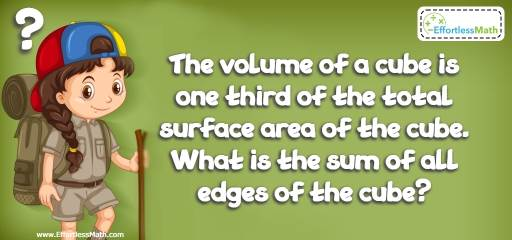# Geometry Puzzle – Critical Thinking 17

If you are a math teacher, you can move beyond the norm in your math classroom and challenge students to think critically with this kind of critical thinking challenge!## Challenge:

The volume of a cube is one-third of the total surface area of the cube. What is the sum of all edges of the cube?

### The Absolute Best Book to Challenge Your Smart Student!

A cube has 12 edges. The formula for the volume and surface area of a cube are:
V = S$$^3$$             SA = 6S$$^2$$
The volume of a cube is one-third of the total surface area of the cube. Thus:
S$$^3= \frac{1}{3}$$6S$$^2$$
Remove S$$^2$$ from both sides and solve for S.
S = $$\frac{1}{3}$$6 = 2
One side of the cube is 2. The sum of all edges is
2 × 12 = 24

The Absolute Best Books to Ace Algebra

### What people say about "Geometry Puzzle – Critical Thinking 17 - Effortless Math: We Help Students Learn to LOVE Mathematics"?

No one replied yet.

X
45% OFF

Limited time only!

Save Over 45%

SAVE $40 It was$89.99 now it is \$49.99